Following are Steps to Make Fund Flow

#### Step 1 Get Balance Sheet of atleast 2 years on Same Excel Sheet in Comparison format

Example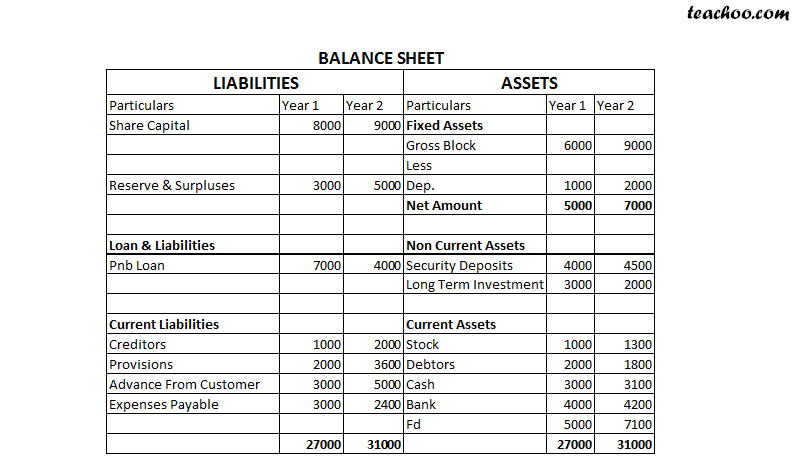#### Step 2 Finding Long Term and Short Term Items in  Balance Sheet

Items In Balance Sheet are of 2 types

Long Term

and

Short Term

#### What are Long Term Items?

All Items of Balance Sheet except Current Assets and Curent Liabilities are Long Term Items

#### What are Short Term Items?

Only Current Assets and Curent Liabilities are Short Term Items

Example

In above Balance Sheet,Figures Colored Red are Short Term Items and those in Black are Long Term Items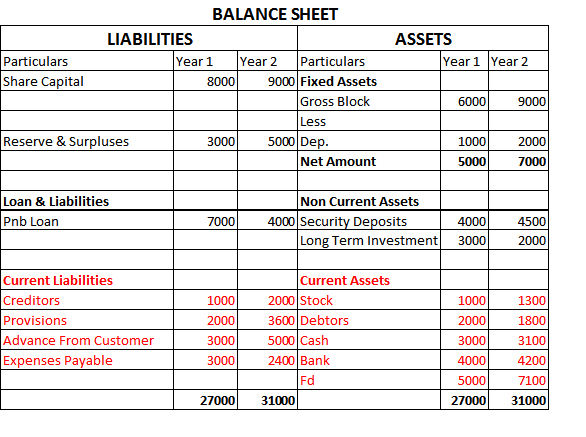#### Step 3 Find Long Term Sources, Long Term Uses and Long Term Surplus/Deficit

How to Determine Sources and Uses of Funds?

 Case Source/ Use If Liability Increase Source If Liability Decrease Use If Assets Increase Use If Assets Decrease Source

Suppose in our Balance Sheet

Following are Long Term Sources and Uses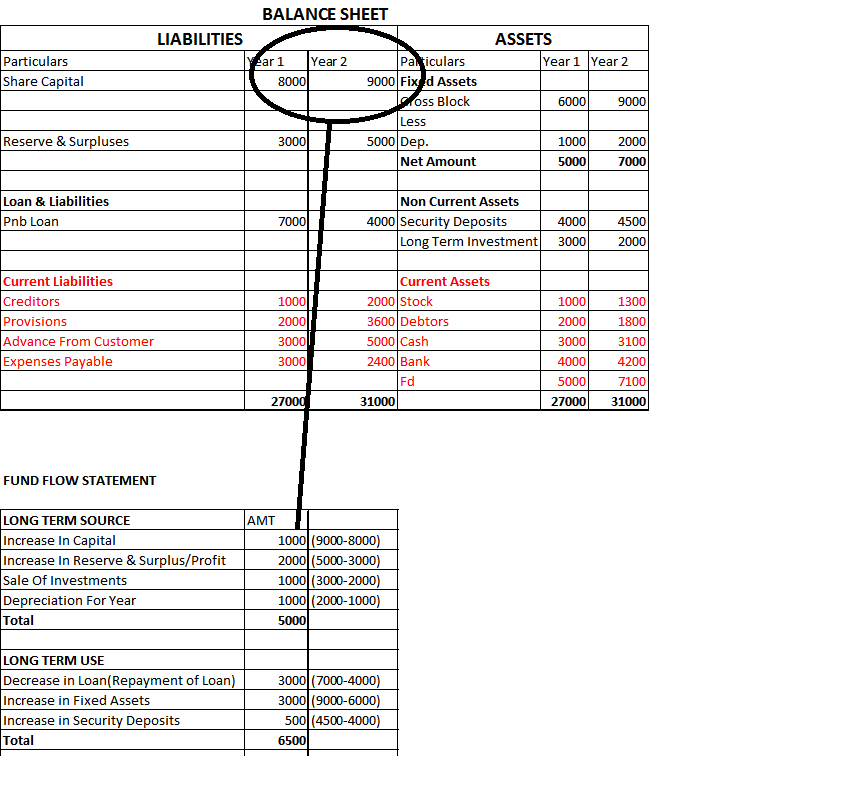Long Term Surplus (Deficit)

Suppose Total Long Term Sources are 10000 and Total Long Term Uses are 7000,

Then Long Term Surplus=3000

Suppose Total Long Term Sources are 10000 and Total Long Term Uses are 11000,

Then Long Term Deficit=1000

In our example above:-Total Long Term Sources are 5000 and Total Long Term Uses are 6500,

Then Long Term Deficit=1500

Step 4 Similarly Find Short Term Sources, Short Term Uses and Short Term Surplus/Deficit

Same Rules are to be followed for Short Term

 If Liability Increase Source If Liability Decrease Use If Assets Increase Use If Assets Decrease Source

Example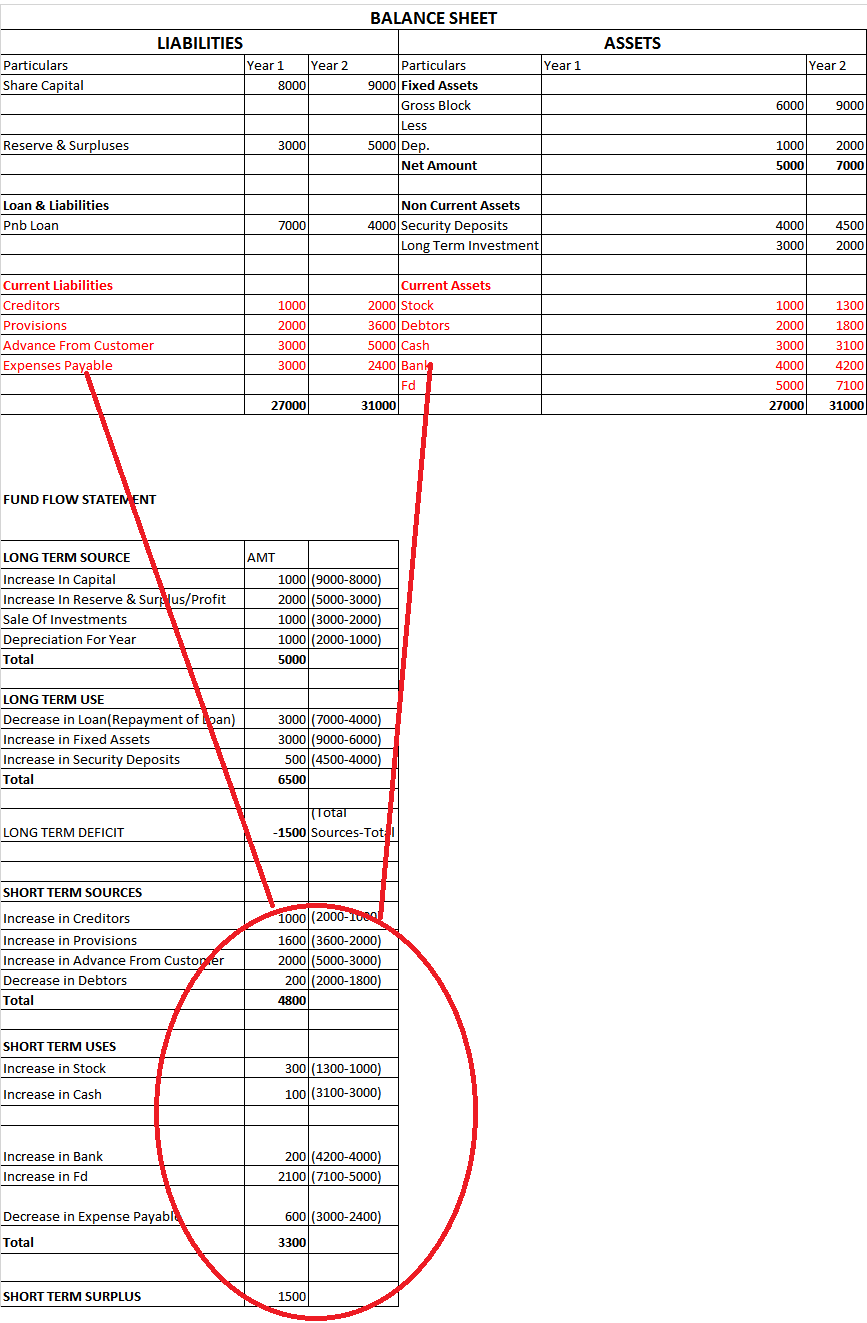Note:-Some people take Total Current Assets and Current Liability to determine Short Term Sources and Used

Example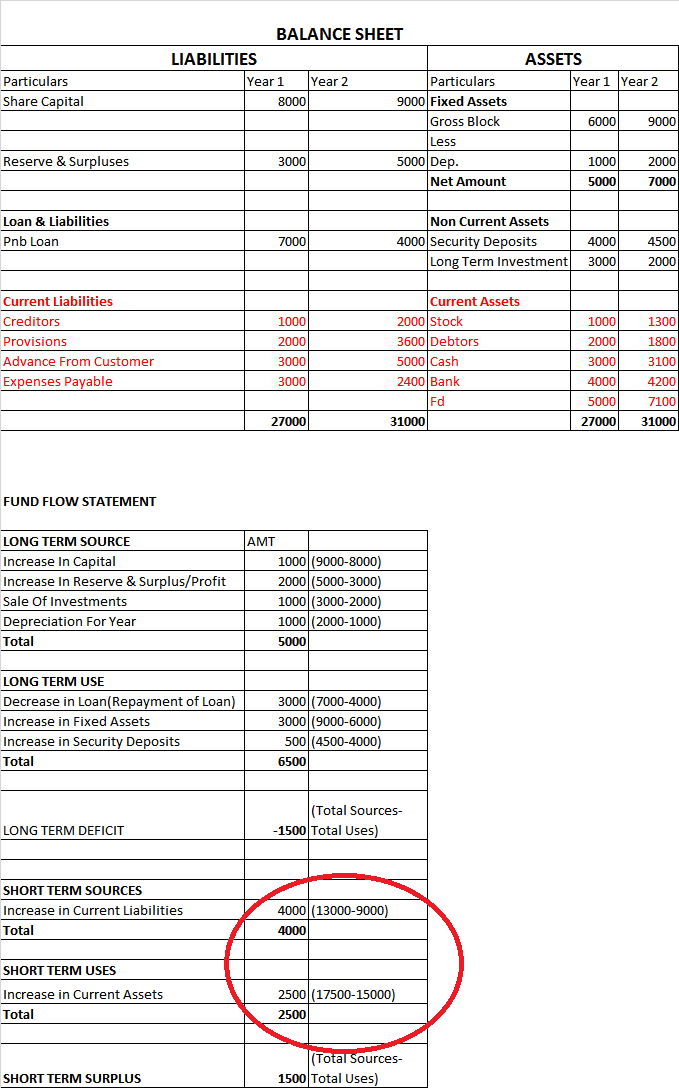#### Step 5 Check by Calculating Net Surplus

Net Surplus is the total of Long Term and Short Term Surplus/ Deficit

If Net Surplus is 0, It means we have prepared Fund Flow Correctly

Suppose there is Long Term Surplus of 400,there will be Short Term Deficit of (400)

Net Surplus=400+(400)=400-400=0

Suppose there is Long Term Deficit of (600),there will be Short Term Surplus of 600

Net Surplus=(600)+600=-600+600=0

If Net Surplus is not coming to 0,it means there is some mistake

Suppose in our Example, Net Surplus=0(-1500+1500) as shown below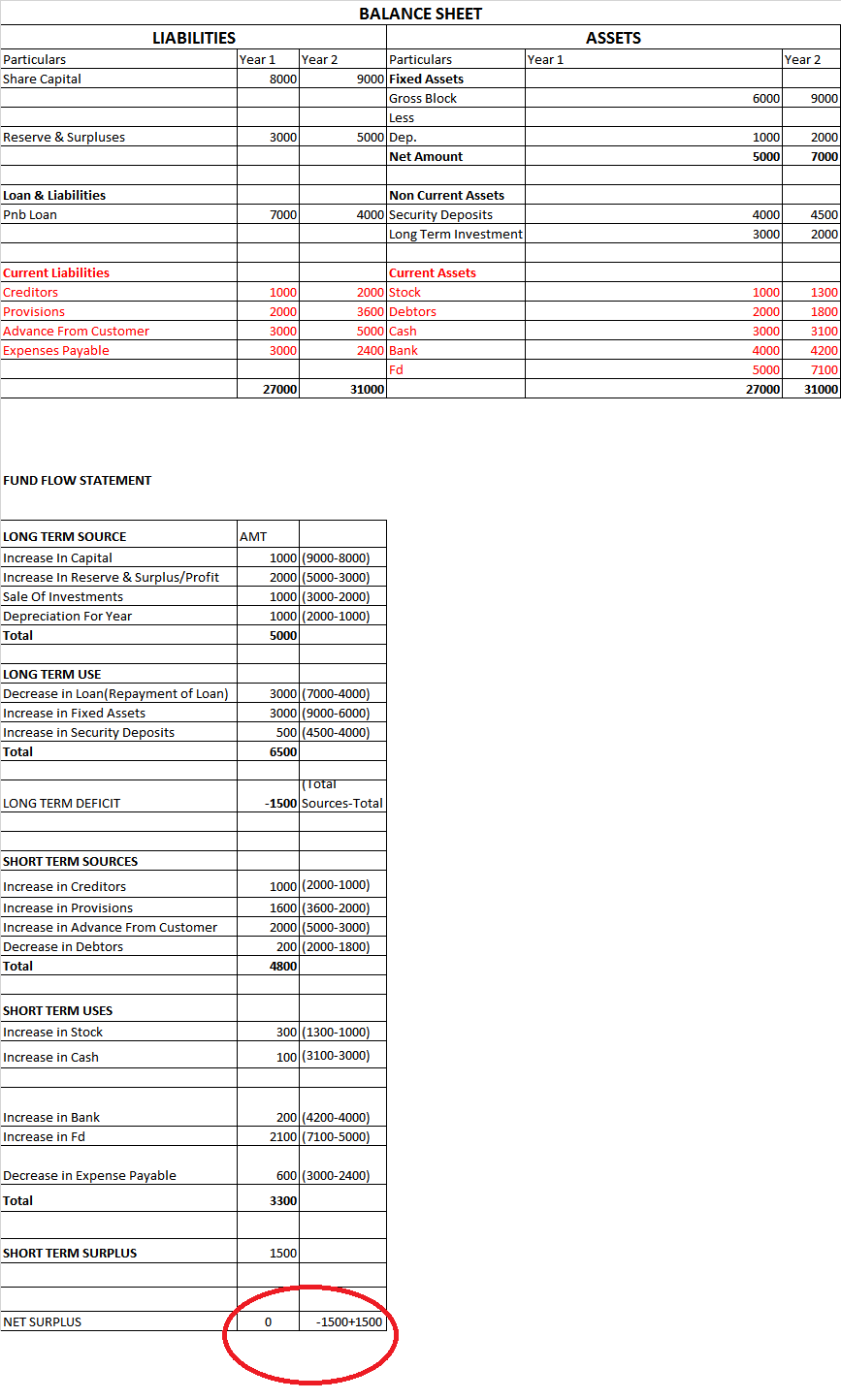This is our Final Fund Flow Statement

1. Accounts and Finance
2. Step 6 Fund Flow and Cash Flow
3. Fund Flow Statement

Fund Flow Statement

Accounts and Finance
Step 6 Fund Flow and Cash Flow